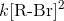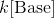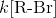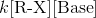Organic Chemistry : Help with Rate Calculations and Kinematics

Example Questions

Example Question #7 : Kinetics And Kinematics

What kinetic equation describes the rate of an E1 process?None of theseExplanation:

Most organic reactions are carried out in multiple steps. The rate equation can be derived from the process that occurs in the slowest step of the mechanism. An E1 reaction's slow step is when a leaving group separates from the hydrocarbon and a carbocation is formed. The only reactant in this step is one molar equivalent of the hydrocarbon. Thus, the rate equation only depends on that substance. The molar equivalent determines the exponent of each reactant in the equation.

Example Question #1 : Help With Rate Calculations And Kinematics

Generally, when compared to the rate of inorganic reactions, the rate of organic reactions is __________.

Faster, because organic compounds contain covalent compounds

Slower, because organic compounds are ionic

Slower, because organic compounds contain covalent bonds

Faster, because organic compounds are ionic